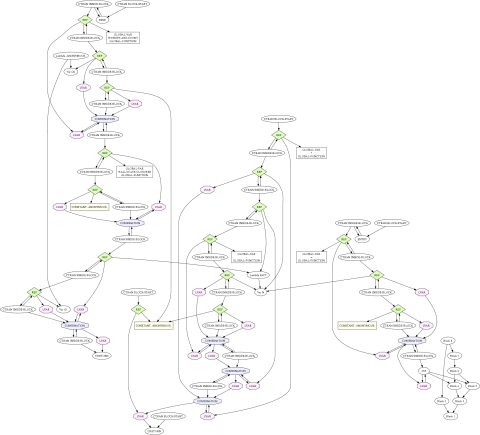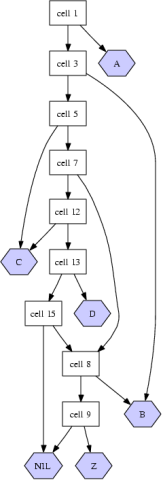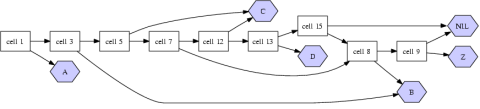CL-DOT is a small package for easily generating dot (a program in the Graphviz suite) output from arbitrary Lisp data.Once again it was time to generate some output suitable for `dot`. Nothing new, stuff that I've done at least four times in three languages (including twice in Lisp). But this time around the data I was going to graph was sufficiently complicated (at least a dozen different kinds of nodes) that it made sense to actually think about it, and not write an unextensible 50-line function to do the work.

There's a protocol of four generic functions, one of which must be defined for every object in the graph (`OBJECT-NODE`) and three others which are used for expressing different relations between objects in the graph (`OBJECT-POINTS-TO`, `OBJECT-POINTED-TO-BY` and `OBJECT-KNOWS-OF`). For example:

``````;; Conses
(defmethod cl-dot:object-node ((object cons))
(make-instance 'cl-dot:node
:attributes '(:label "cell \\N"
:shape :box)))
(defmethod cl-dot:object-points-to ((object cons))
(list (car object)
(make-instance 'cl-dot:attributed
:object (cdr object)
;; Make the CDR edges more important than the
;; CAR edges
:attributes '(:weight 3))))
;; Symbols
(defmethod cl-dot:object-node ((object symbol))
(make-instance 'cl-dot:node
:attributes `(:label ,object
:shape :hexagon
:style :filled
:color :black
:fillcolor "#ccccff")))
``````

To generate a graph object for your data, call `GENERATE-GRAPH`. From the graph object you can either generate dot-format output to some stream with `PRINT-GRAPH`, or call `dot` directly on the data with `DOT-GRAPH`. For example:

``````(let* ((data '(a b c #1=(b z) c d #1#))
(graph (cl-dot:generate-graph data)))
(cl-dot:dot-graph graph "/tmp/test1ps"))
``````You can also specify attributes for the whole graph:

``````(let* ((data '(a b c #1=(b z) c d #1#))
(graph (cl-dot:generate-graph data '(:rankdir "LR"))))
(cl-dot:dot-graph graph "/tmp/test2ps"))
``````